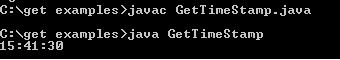Tutorials

Get Time Stamp

In the example given below we will learn how to display current time. getTime() method returns the number of milliseconds represented by Date object that will be used as argument to create object of java.sql.Time class.

In the example given below we will learn how to display current time. getTime() method returns the number of milliseconds represented by Date object that will be used as argument to create object of java.sql.Time class.

Get Time Stamp

In the example given below we will learn how to display current time. getTime() method returns the number of milliseconds represented by Date object that will be used as argument to create object of java.sql.Time class.

Here is the code:

 public class GetTimeStamp {   public static void main(String[] args) {   java.util.Date time = new java.util.Date();   System.out.println(new java.sql.Time(time.getTime()));    } }

Output will be displayed as: